# MCMC

### Introduction

Markov Chain Monte-Carlo (MCMC) is a method of sampling from a distribution, when it is difficult to sample from the distribution directly. It can be used with Monte-Carlo Integration for numerical calculation of integrals.

It explores the state space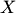using a Markov Chain mechanism, and produces samples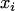which mimic samples drawn from the distribution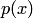directly. We assume that we can evaluateup to a normalizing constant, but it is difficult to draw samples from it.

Let us consider the situation when the number of possible states in a Markov chain is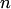. Then we can choose an initial distribution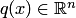and have a transition matrix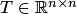. Following the transition matrix at the first step, we get the distribution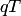. If we repeat the process several times and it converges to a distribution, we say it converged to an invariant distribution. The process converges if the matrix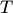has properties of irreducibility and aperiodicity.

Definition 1. A transition matrixis irreducible if for any state of the Markov chain there is a positive probability of visiting all other states.

Definition 2. A transition matrixis aperiodical if the Markov chain does not get trapped into cycles.

A sufficient condition for the transition matrix to satisfy these requirenments is the reversibility condition: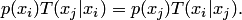In this casehere is the invariant distribution. Indeed, summing both sides overgives us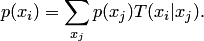In continuous state spaces the transition matrixbecomes an integral kernel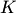andbecomes the corresponding eigenfunction.

MCMC samples are organized in a way that the desiredis the invariant distribution:

### The Metropolis-Hastings algorithm

Let us have a proposal distribution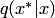and invariant distribution. An MH step involves sampling from the proposal distribution a candidate value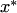. The Markov chain then moves towardswith acceptance probability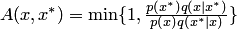, otherwise it remains at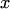.

Initialize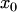;
FOR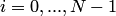Sample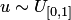from the uniform distribution.
Sample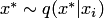from the proposal distribution.
If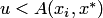then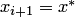else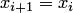.
END FOR.

If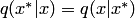the MH algorithm transforms to the Metropolis algorithm.

### Integration using MCMC

To calculate the integral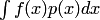it is possible to sample from the uniform distribution and calculate the integral using Monte-Carlo integration. But MCMC method of sampling frommay lead to much better convergence. First one needs to perform burn-in state, when a Markov chain finds a way to sample from a distribution close tousing MH algorithm. Then search for the distribution continues, but the sum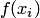is calculated. The resulting approximation of the integral is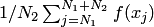, where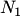is the length of the burn-in stage, and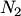is the length of the sampling stage.

MCMC is also used to solve optimization problems like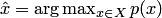by applying a so-called simulated annealing technique.

### Bibliography

• Metropolis, N., Rosenbluth, A.W., Rosenbluth, M.N., Teller, A.H., Teller, E. Equations of State Calculations by Fast Computing Machines. Journal of Chemical Physics, 21(6): 1087–1092 (1953).
• Hastings, W.K. Monte Carlo sampling methods using Markov chains and their applications. Biometrika, 57(1):97–109 (1970).
• Christophe Andrieu et al. An Introduction to MCMC for Machine Learning, Machine Learning, 50: 5–43 (2003).